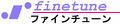for HP 42S
Pt100 - Pt100-1997 Platinum RTD (Resistance Temperature Detectors) Resistance V.S. Termperature function

Usage
```Resistance → Temperature conversion [SOLVER] [Pt100] Resistance [R] [Temp] Temperature → Resistance conversion [SOLVER] [Pt100] Temperature [Temp] [R] ```
Variables
```MEM "R" : resistance [Ω] MEM "Temp" : temperature [°C] ```
Callendar-Van Dusen equation: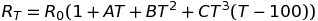```RT : Resistance [Ω] at temperature T [°C] R0 : Resistance [Ω] at 0 °C T : Temperature in °C A : 3.9083 × 10-3 B : -5.775 × 10-7 C : -4.183 × 10-12 | -200 ≤ T < 0 : 0 | 0 ≤ T ≤ +650 The equation is applicable for -200 ≤ T ≤ +650 ``` Pt100 Resistance V.S. Temperature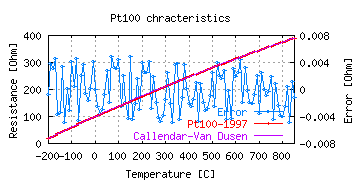R : Resistance Ω T : Temperature ℃

Program list for HP 42S (Rev.1.00 Jan. 24, 2010)
"Pt100" : Pt100-1997 RTD function
 To be used in the SOLVER ``````01 LBL "Pt100" 02 MVAR "Temp" 03 MVAR "R" 04 RCL "Temp" 05 ENTER 06 ENTER 07 ENTER 08 X>0? 09 100 10 100 11 - 12 -4.183E-12 13 × 14 × 15 -5.775E-7 16 + 17 × 18 3.9083e-3 19 + 20 × 21 1 22 + 23 100 24 × 25 RCL- "R" 26 .END. ``````

#### APPENDIX

Program library for the HP 42S
• ABC2XY - Triangulation, tree known distance to the coordinates conversion
• AVR - Adjustable voltage regulator (such as LM317 or TL431) solver program
• BPFFL - Center frequency and Q of a Fliege band-pass-filter
• BPFMF - Gain coefficent, center frequency and Q of a multiple-feedback band-pass-filter
• E24 - Find nearest fraction(s) in the E24 numbers
• eW - exponent of Lambert W function
• Factr - Factor a positive integer
• Frac - Fractional approximation
• LCM/GCD - LCM and GCD of two positive integers
• LCR - Resonant frequency of a LC resonator
• LPFMF - Gain coefficent, center frequency and Q of a multiple-feedback low-pass-filter
• L• - Inductanse of a straight conductor of circular section
• L- - Inductanse of a straight conductor of thin flat section
• NTC10K - NCP21XV103 NTC thermistor Temperature V.S. Resistance function to solve
• OST - SOLVE alternative, numerical solution of a lenear equation using Ostrowski's method
• RI - Rational interpolation
• Pt100 - Pt100-1997 Temperature V.S. Resistance function to solve
• μZw, μwZ - Synthesize/Analyze microstrip transmission line
• Vn - Input refered voltage noise of an operational amplifier
• Z→S - Impedance Z to S11 scattering parameter conversion
• ZdSL - Differential impedance of an edge coupled stripline solver program

REFERENCES
 JIS C 1604-1997
 IEC 60751 Amd.2-1995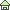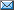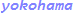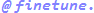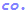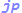Copyright (c) HOSODA Takayuki. All rights reserved.